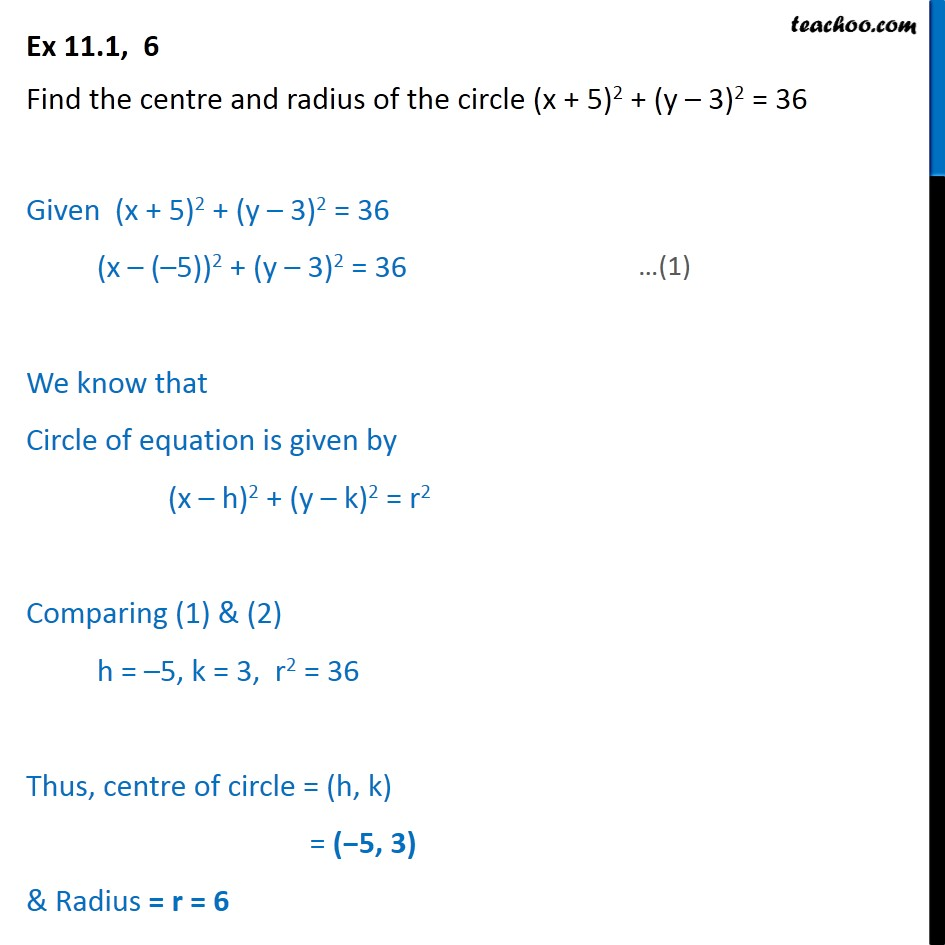Ex 10.1

Chapter 10 Class 11 Conic Sections
Serial order wiseLearn in your speed, with individual attention - Teachoo Maths 1-on-1 Class

### Transcript

Ex 10.1, 6 Find the centre and radius of the circle (x + 5)2 + (y 3)2 = 36 Given (x + 5)2 + (y 3)2 = 36 (x ( 5))2 + (y 3)2 = 36 We know that Circle of equation is given by (x h)2 + (y k)2 = r2 Comparing (1) & (2) h = 5, k = 3, r2 = 36 Thus, centre of circle = (h, k) = ( 5, 3) & Radius = r = 6